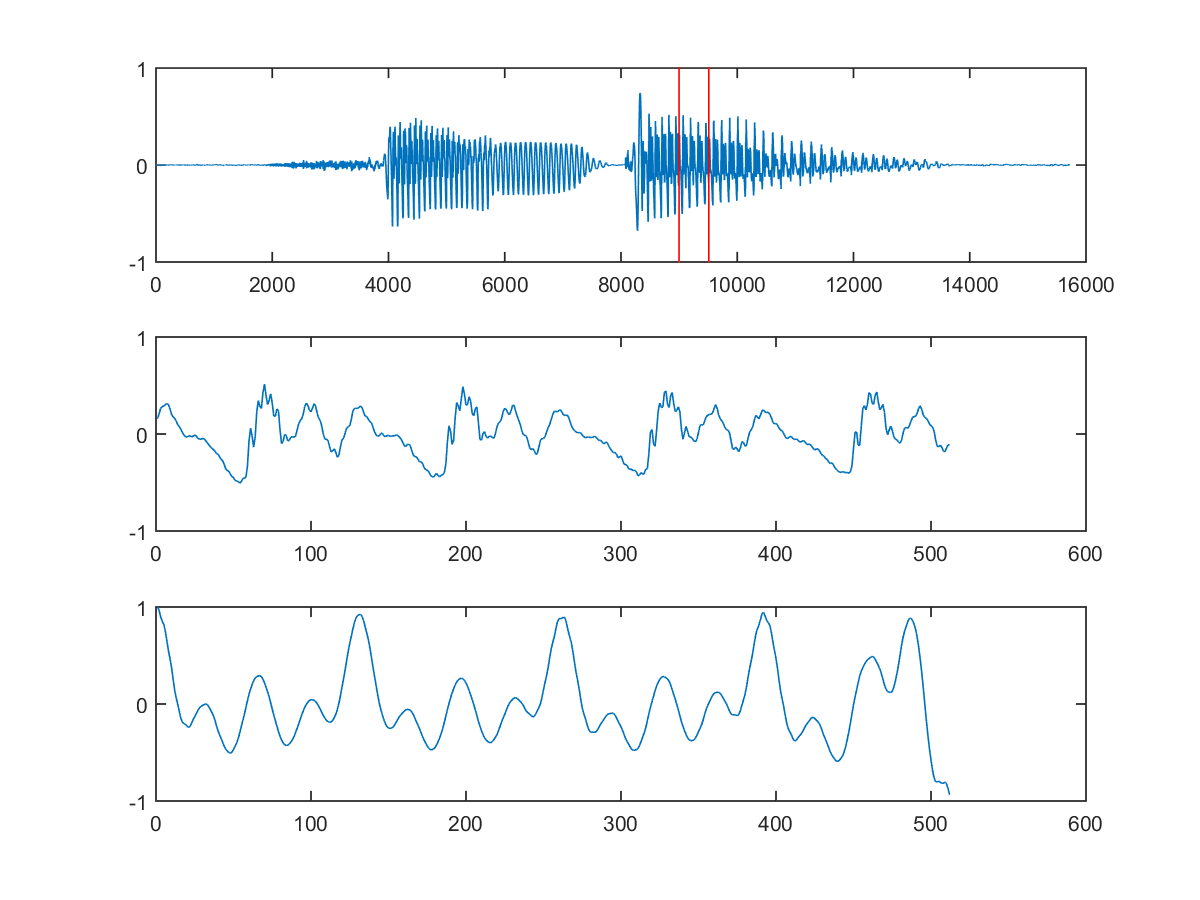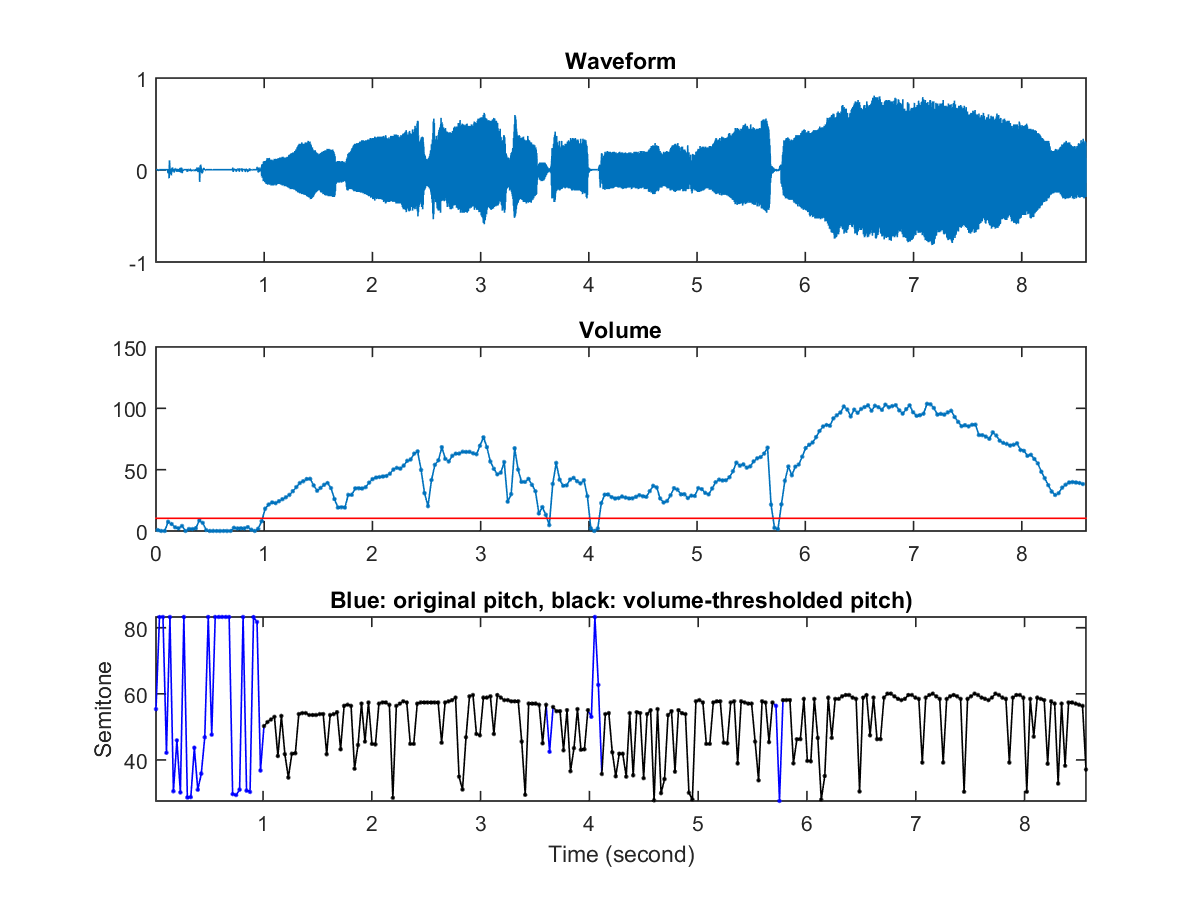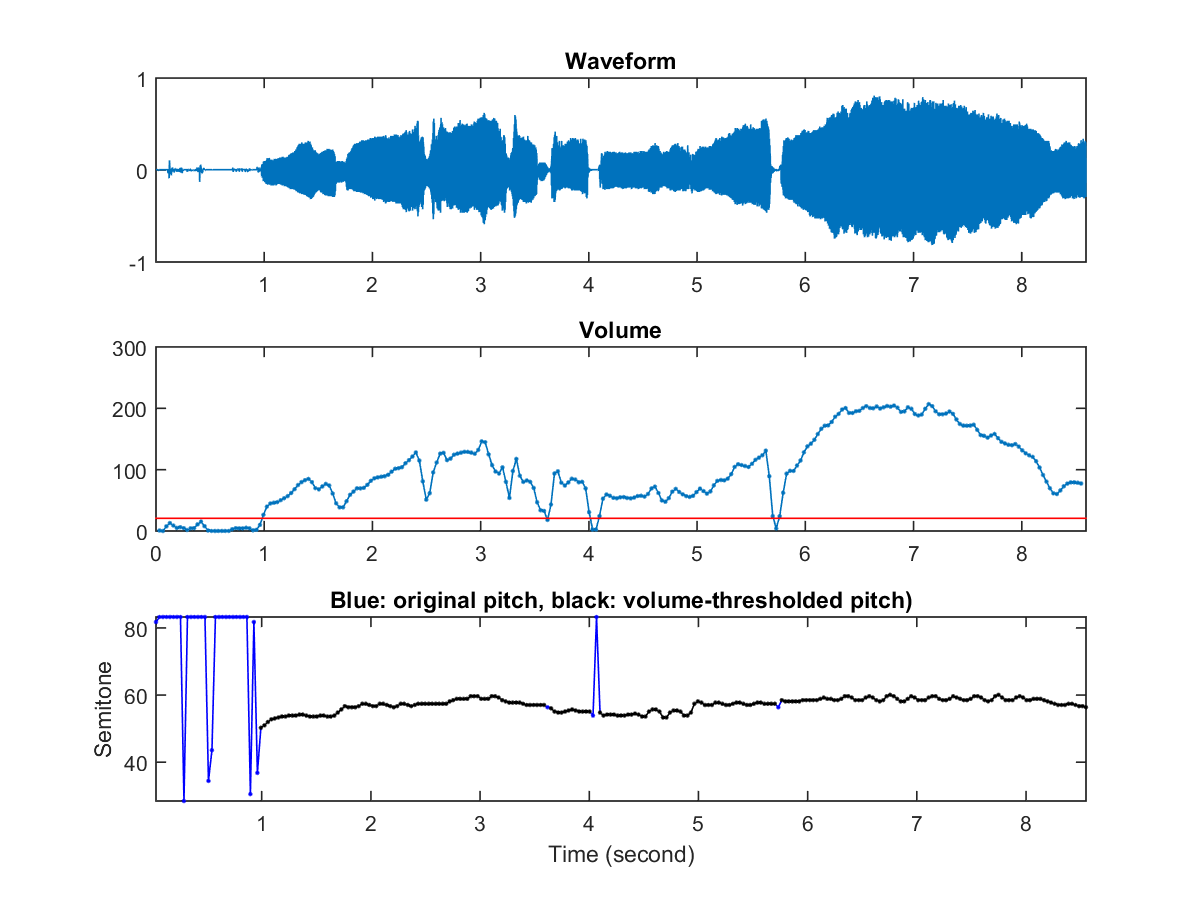## 7-3 Time-domain: PDF: NSDF

The range of ACF is usually not known in advance. To limit the range of ACF to [-1, 1], we can use the following NSDF (normalized squared difference function) formula: $$nsdf(\tau)=\frac{2\sum s(i)s(i+\tau)}{\sum s^2(i)+\sum s^2(i+\tau)}$$ All the summations in the above equation should have the same lower and upper bounds. The range of NSDF is [-1, 1] due to the following inequality: $$-1 \leq \frac{2xy}{x^2+y^2} \leq 1$$ If the selected pitch point is $\tau=\tau_0$, then we define the clarity of this frame is $$clarity=acf(\tau_0)$$ A higher clarity indicates the frame is closer to a pure periodic waveform. On the other hand, a lower clarity indicates the frame is less periodic, which is likely to be caused by unvoiced speech or silence. The following is a typical example:

Example 1: frame2nsdf01.mwaveFile='sunday.wav'; au=myAudioRead(waveFile); index1=9000; frameSize=512; index2=index1+frameSize-1; frame=au.signal(index1:index2); maxShift=length(frame); opt=frame2pdf('defaultOpt'); opt.pdf='nsdf'; opt.maxShift=length(frame); opt.method=1; nsdf=frame2pdf(frame, opt); subplot(3,1,1); plot(au.signal); line(index1*[1 1], [-1 1], 'color', 'r'); line(index2*[1 1], [-1 1], 'color', 'r'); subplot(3,1,2); plot(frame); subplot(3,1,3); plot(nsdf);The following example uses NSDF to perform pitch tracking:

Example 2: ptByNsdf01.mwaveFile='soo.wav'; opt=pitchTrackBasic('defaultOpt'); opt.frameDuration=32; % Duration (in ms) of a frame opt.overlapDuration=0; % Duration (in ms) of overlap opt.frame2pitchOpt.pdf='nsdf'; opt.frame2pitchOpt.method=1; showPlot=1; pitch=pitchTrackBasic(waveFile, opt, showPlot);We can increase the frame size to reduce pitch-halving errors:

Example 3: ptByNsdf03.mwaveFile='soo.wav'; opt=pitchTrackBasic('defaultOpt'); opt.frameDuration=32*2; % Duration (in ms) of a frame opt.overlapDuration=32; % Duration (in ms) of overlap opt.frame2pdfOpt.pdf='nsdf'; opt.frame2pdfOpt.method=3; showPlot=1; pitch=pitchTrackBasic(waveFile, opt, showPlot);Reference: McLeod, Philip, and Geoff Wyvill. "A smarter way to find pitch." Proceedings of International Computer Music Conference, ICMC. 2005.
Audio Signal Processing and Recognition (音訊處理與辨識)